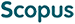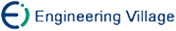Lin Deng, Cui Tao, Leng Wei, Zhang Linbo. An Efficient Parallel Spectral Element Scheme for Solving Seismic Wave Equation[J]. Journal of Computer Research and Development, 2016, 53(5): 1147-1155. doi: 10.7544/issn1000-1239.2016.20148440
 Citation: Lin Deng, Cui Tao, Leng Wei, Zhang Linbo. An Efficient Parallel Spectral Element Scheme for Solving Seismic Wave Equation[J]. Journal of Computer Research and Development, 2016, 53(5): 1147-1155. doi: 10.7544/issn1000-1239.2016.20148440# An Efficient Parallel Spectral Element Scheme for Solving Seismic Wave Equation• Numerical simulation of seismic waves plays an essential role in seismology and seismic exploration. We propose here an efficient parallel spectral element scheme for seismic wave equation with perfectly matched layer (PML). PML is integrated into the seismic wave equation to absorb out-going waves and mimic unbounded domain. Ulteriorly, to enable adapting complex topography and explicit time stepping, the spectral element method (SEM) is used to discretize seismic wave equation with PML, which results in a spectral element scheme. In addition, we demonstrate that element stiffness matrices can be decomposed, which can be used to greatly reduce the storage of stiffness matrix and accelerate stiffness matrix-vector multiplication and thus remarkably speed up the scheme and cut down memory cost. Furthermore, we study several spectral element schemes known and show that our scheme is superior to others in both calculation and storage. Combined with parallel technique, an efficient parallel spectral element solver for seismic wave equation with PML is present. Numerical experiments show that our scheme is correct, well stronglyweakly scalable and of good adaptation to complex topography.
•/DownLoad:  Full-Size Img  PowerPoint